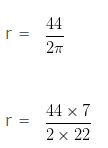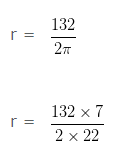# Find Diameter from Circumference

Diameter of the circle

A line segment which passes through the center of a circle, and both of whose endpoints lie on the circumference of the circle is called the Diameter of a circle.
Diameter of circle is equal to twice of the length of its radius.
Diameter of the circle = 2r

Circumference of the circle

Circumference of a circle is the measure of the length of the circle.
Circumference of the circle = 2πr

### Questions Based on Finding Diameter of a circle when circumference is given

Question 1

Find the diameter of the circle whose circumference is 44 cm?

Explanation

Let, the radius of the given circle be r cm

As we know that,

Circumference of the circle = 2πr cm

Given,

Circumference of a cicle = 44cm

Therefore,

2πr = 44 (from above)r = 7cm

Diameter of the circle = 2r

= (2 x 7)cm

= 14cm

Hence, the Diameter of the circle is 14 cm

Question 2

Find the diameter of the circle whose circumference is 132 cm?

Explanation

Let, the radius of the given circle be r cm

As we know that,

Circumference of the circle = 2πr cm

Given,

Circumference of a cicle = 132cm

Therefore,

2πr = 132 (from above)r = 21cm

Diameter of the circle = 2r

= (2 x 21)cm

= 42cm

Hence, the Diameter of the circle is 42 cm